### IMO Shortlist 2012 problem G7

Kvaliteta:
Avg: 0,0
Težina:
Avg: 9,0
Let$ABCD$ be a convex quadrilateral with non-parallel sides$BC$ and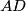$AD$. Assume that there is a point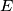$E$ on the side$BC$ such that the quadrilaterals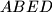$ABED$ and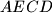$AECD$ are circumscribed. Prove that there is a point$F$ on the side$AD$ such that the quadrilaterals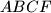$ABCF$ and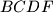$BCDF$ are circumscribed if and only if$AB$ is parallel to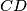$CD$.
Izvor: Međunarodna matematička olimpijada, shortlist 2012